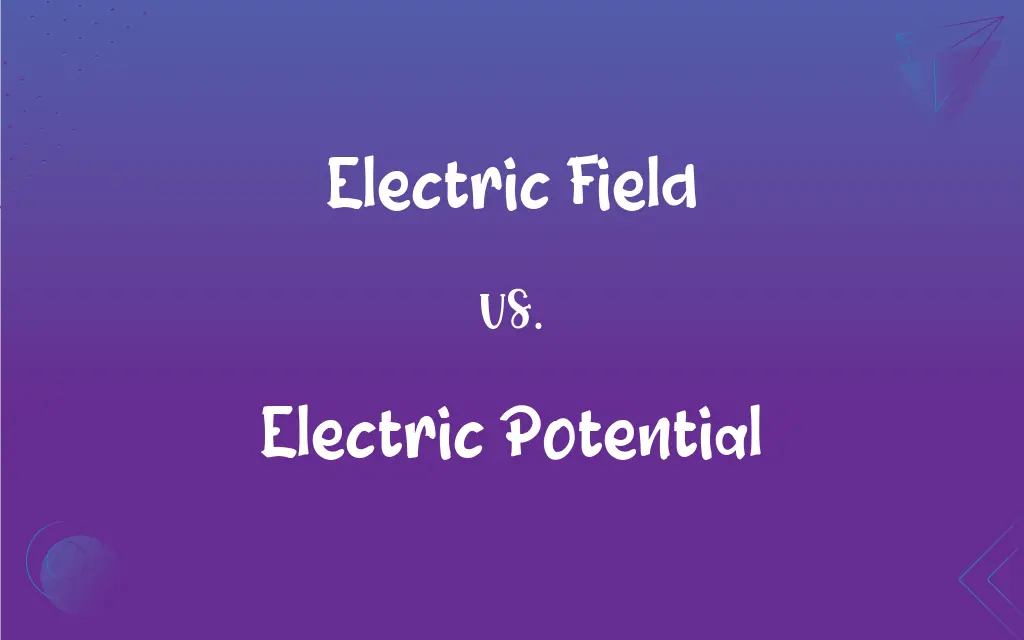# Electric Field vs. Electric Potential: What's the Difference?Edited by Sumera Saeed || By Sawaira Riaz || Published on November 2, 2023
Electric Field represents the force per unit charge acting on a charge, while Electric Potential signifies the work done per unit charge to move a charge to a point.## Key Differences

An Electric Field represents the force acting on a unit positive charge placed in the field. It gives direction and magnitude of the force. On the other hand, Electric Potential is a scalar quantity that denotes the work done to move a unit charge from infinity to a specific point in the field without acceleration.
The unit of Electric Field is Newton per Coulomb (N/C) or Volt per meter (V/m) in the SI system. Conversely, the Electric Potential has a unit of Volt (V) representing energy per unit charge.
Electric Field is often represented by vector lines pointing in the direction of the force. The Electric Potential, being scalar, doesn't have a direction but represents points of equal potential as equi-potential surfaces or lines.
The Electric Field is the negative gradient (rate of change) of the Electric Potential. So, in simple terms, the Electric Field points in the direction where the Electric Potential decreases most rapidly.
A charge in an Electric Field experiences a force, while the work done in moving a charge within an Electric Potential difference results in potential energy for that charge.

## Comparison Chart

### Definition

Force per unit charge
Work done per unit charge

### Unit

Newton per Coulomb (N/C) or Volt per meter (V/m)
Volt (V)

Vector
Scalar

### Representation

Vector lines
Equi-potential surfaces/lines

### Relation to other

Electric Potential is integrated from Electric Field

## Electric Field and Electric Potential Definitions

#### Electric Field

Electric Field lines point from positive to negative charges.
Using field lines, one can visualize the strength and direction of the Electric Field.

#### Electric Potential

Electric Potential represents energy per unit charge.
A point with higher Electric Potential has more electric potential energy.

#### Electric Field

An Electric Field is the space where electric forces act.
Near a charged object, the Electric Field is stronger.

#### Electric Potential

Electric Potential is a scalar quantity.
Electric Potential values at specific points don't have a direction, unlike Electric Field.

#### Electric Field

An Electric Field represents force experienced by a unit positive charge.
The Electric Field around a positive charge pushes away other positive charges.

#### Electric Potential

Electric Potential is the work done to move a unit charge to a point.
Higher Electric Potential regions require more work to move a charge to.

#### Electric Field

Electric Field can induce motion in charges present within it.
When a charge is placed in an Electric Field, it experiences a force.

#### Electric Potential

Electric Potential is the integral of the Electric Field.
Integrating the Electric Field over a distance gives the Electric Potential difference.

#### Electric Field

The strength of an Electric Field is directly proportional to the charge producing it.
A charge with greater magnitude produces a stronger Electric Field.

#### Electric Potential

Electric Potential differences cause the movement of charges.
Batteries provide an Electric Potential difference that drives current in circuits.

## FAQs

#### What does the Electric Field denote?

Electric Field denotes the force experienced by a unit positive charge placed in the field.

#### What is Electric Potential's significance?

Electric Potential signifies the work done to move a unit charge to a specific point in an Electric Field.

#### Can you have an Electric Potential without an Electric Field?

No, an Electric Potential difference implies the presence of an Electric Field.

#### How is the direction of the Electric Field determined?

The Electric Field direction is where a positive test charge would experience a force.

#### Is Electric Field a vector?

Yes, Electric Field is a vector quantity, having both magnitude and direction.

#### Can charges move against an Electric Potential difference?

Yes, but external work is needed to move charges against an Electric Potential difference.

#### What unit measures Electric Potential?

Electric Potential is measured in volts (V).

#### How are Electric Field lines drawn?

Electric Field lines are drawn from positive charges to negative charges.

#### What happens to a charge in a region of high Electric Potential?

A charge in a high Electric Potential region possesses higher potential energy.

#### What does a stronger Electric Field indicate?

A stronger Electric Field indicates a larger force experienced by a charge placed in that field.

#### Why is Electric Potential important in circuits?

Electric Potential differences in circuits drive the movement of charges, causing electric current.

#### What does zero Electric Potential mean?

Zero Electric Potential often refers to a reference point, like the Earth, against which other potentials are measured.

#### How does distance affect Electric Field and Electric Potential?

As distance from a charge increases, both Electric Field and Electric Potential typically decrease.

#### Can Electric Field and Electric Potential be negative?

Yes, both Electric Field and Electric Potential can be negative, depending on charge and reference direction.

#### Can two points have the same Electric Potential?

Yes, points with the same Electric Potential form equi-potential surfaces or lines.

#### Can Electric Field exist in a vacuum?

Yes, Electric Field can exist in a vacuum.

#### What causes an Electric Field?

Electric charges, whether stationary or in motion, produce an Electric Field.

#### What's the effect of a charge in an Electric Field?

A charge in an Electric Field experiences a force and may move if not restrained.

#### How are Electric Field and Electric Potential related?

Electric Field is the negative gradient of Electric Potential.

#### Does Electric Potential have a direction?

No, Electric Potential is scalar and does not have a direction.